# Engineering Mechanics - Structural Analysis

## Why Engineering Mechanics Structural Analysis?

In this section you can learn and practice Engineering Mechanics Questions based on "Structural Analysis" and improve your skills in order to face the interview, competitive examination and various entrance test (CAT, GATE, GRE, MAT, Bank Exam, Railway Exam etc.) with full confidence.

## Where can I get Engineering Mechanics Structural Analysis questions and answers with explanation?

IndiaBIX provides you lots of fully solved Engineering Mechanics (Structural Analysis) questions and answers with Explanation. Solved examples with detailed answer description, explanation are given and it would be easy to understand. All students, freshers can download Engineering Mechanics Structural Analysis quiz questions with answers as PDF files and eBooks.

## Where can I get Engineering Mechanics Structural Analysis Interview Questions and Answers (objective type, multiple choice)?

Here you can find objective type Engineering Mechanics Structural Analysis questions and answers for interview and entrance examination. Multiple choice and true or false type questions are also provided.

## How to solve Engineering Mechanics Structural Analysis problems?

You can easily solve all kind of Engineering Mechanics questions based on Structural Analysis by practicing the objective type exercises given below, also get shortcut methods to solve Engineering Mechanics Structural Analysis problems.

### Exercise :: Structural Analysis - General Questions

• Structural Analysis - General Questions
1.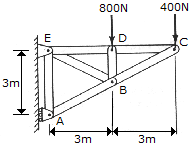Determine the force in each member of the truss and indicate whether the members are in tension or compression.

 A. CB = 447 N C, CD = 200 N T, DB = 800 N C, DE = 200 N T, BE = 447 N T, BA = 894 N C, AE = 800 N T B. CB = 447 N T, CD = 200 N C, DB = 800 N T, DE = 200 N C, BE = 447 N C, BA = 894 N T, AE = 800 N C C. CB = 894 N T, CD = 800 N C, DB = 800 N T, DE = 800 N C, BE = 894 N C, BA = 1789 N T, AE = 800 N C D. CB = 894 N C, CD = 800 N T, DB = 800 N C, DE = 800 N T, BE = 894 N T, BA = 1789 N C, AE = 800 N T

Explanation:

No answer description available for this question. Let us discuss.

2.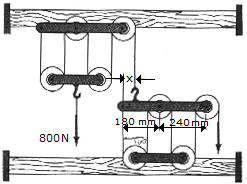The principles of a differential chain block are indicated schematically in the figure. Determine the magnitude of force P needed to support the 800-N force. Also compute the distance x where the cable must be attached to bar AB so the bar remains horizontal. All pulleys have a radius of 60 mm.

 A. P = 80 N, x = 240 mm B. P = 80 N, x = 180 mm C. P = 40 N, x = 180 mm D. P = 40 N, x = 240 mm

Explanation:

No answer description available for this question. Let us discuss.

3.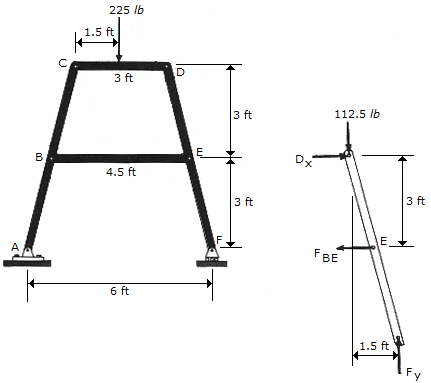Determine the magnitude of the forces in pins B and D of the four-member frame.

 A. B = 503 lb, D = 225 lb B. B = 225 lb, D = 503 lb C. B = 56.3 lb, D = 125.8 lb D. B = 125.8 lb, D = 112.5 lb

Explanation:

No answer description available for this question. Let us discuss.

4.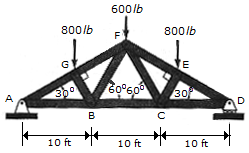Determine the force in members FF, FB, and BC of the Fink truss and indicate whether the members are in tension or compression.

 A. BF = 693 lb T, FG = 1800 lb C, BC = 1212 lb T B. BF = 8660 lb C, FG = 3600 lb C, BC = 1212 lb T C. BF = 3810 lb T, FG = 3600 lb C, BC = 1212 lb T D. BF = 1732 lb T, FG = 2400 lb C, BC = 1212 lb T

Explanation:

No answer description available for this question. Let us discuss.

5.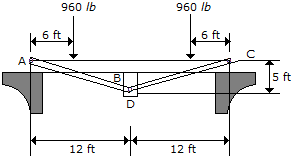The floor beams AB and BC are stiffened using the two tie rods CD and AD. Determine the force along each rod. Assume the three contacting members at B are smooth and the joints at A, C, and D are pins.

 A. T = 480 lb B. T = 520 lb C. T = 1248 lb D. T = 1152 lb